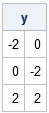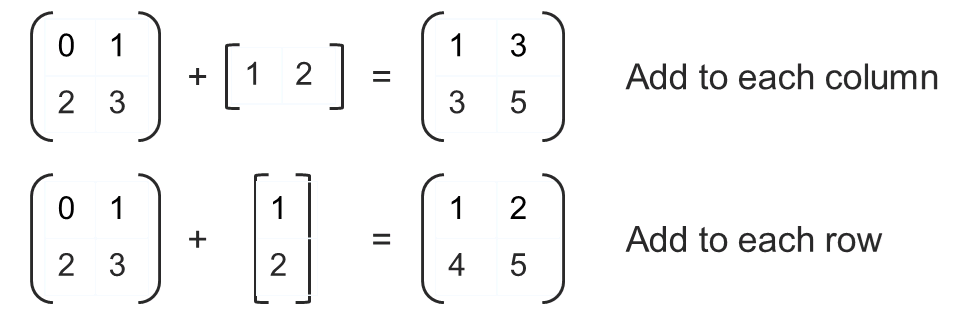The SAS/IML language enables you to perform matrix-vector computations. However, it also provides a convenient "shorthand notation" that enables you to perform elementwise operation on rows or columns in a natural way.

You might know that the SAS/IML language supports subscript reduction operators to compute basic rowwise or columnwise quantities. For example, if X is a matrix, you can easily compute the mean of each column with the expression X[:, ]. The result is a vector with the same number of columns as X.

But did you know that you can immediately use that result to center the matrix X? In other words, if you want to subtract the mean of each column from each column you don't need to write a loop, you simply use the following shorthand notation:

```proc iml; x = {1 2, 3 0, 5 4}; mean = x[:, ]; /** vector that contains the mean of each column **/ y = x - mean; /** subtract mean[j] from column j, j=1,2 **/ print y;```### Operations on Rows and Columns

The SAS/IML language supports shorthand notation for elementwise addition, subtraction, multiplication, division, and exponentiation (raising an element to a power). If A is an n x p matrix, then you can perform operations on columns by using a 1 x p vector. Similarly, you can perform operations on rows by using an n x 1 vector. For example, the following graphic summarizes the addition operations on rows and columns for 2 x 2 matrices:The same rules apply to all of the elementwise operations, as shown by the following statements:

```x = {1 2, 3 4};   /** elementwise column operations **/ u = {1 2}; a = x + u; /** add u[j] to column j **/ s = x - u; /** subtract u[j] from column j **/ m = x # u; /** multiply column j by u[j] **/ d = x / u; /** divide column j by u[j] **/ p = x ## u; /** raise column j to the power u[j]**/ print x u, a s, m d, p;   /** elementwise row operations **/ v = {1, 2}; a = x + v; /** add v[i] to row i **/ s = x - v; /** subtract v[i] from row i **/ m = x # v; /** multiply row i by v[i] **/ d = x / v; /** divide row i by v[i] **/ p = x ## v; /** raise row i to the power v[i]**/ print x v, a s, m d, p;```

Further examples of row and column operations are included in Chapter 2 of my book Statistical Programming with SAS/IML Software, which is available as a free PDF document from my SAS Press author page.

Share